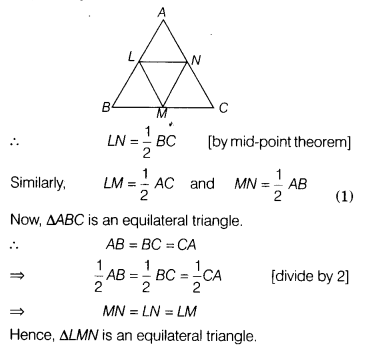# ABC is an equilateral triangle and L, M and N are the mid-points of the sides AB, BC and CA,

ABC is an equilateral triangle and L, M and N are the mid-points of the sides AB, BC and CA, respectively. Prove that ∆LMN is an equilateral triangle.

Given, ∆ABC is an equilateral triangle and L, M, N are the mid-points of the sides AB, BC and CA respectively.
To prove To prove ∆LMN is an equilateral triangle.
Since, L and N are the mid-points of AB and AC, respectively.